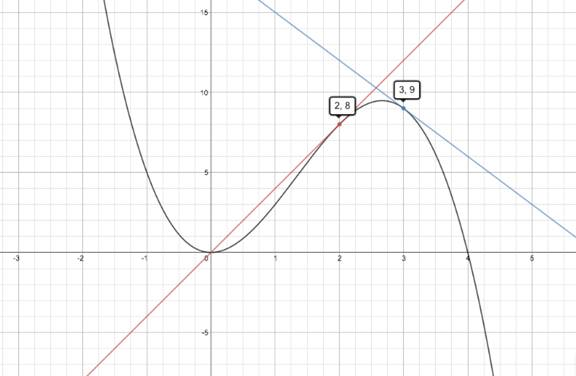# The equation of the tangent line to the curve G ( x ) = 4 x 2 − x 3 at the points ( 2 , 8 ) and ( 3 , 9 ) .### Single Variable Calculus: Concepts...

4th Edition
James Stewart
Publisher: Cengage Learning
ISBN: 9781337687805### Single Variable Calculus: Concepts...

4th Edition
James Stewart
Publisher: Cengage Learning
ISBN: 9781337687805

#### Solutions

Chapter 2.6, Problem 26E

a.

To determine

## To find:The equation of the tangent line to the curve G(x)=4x2−x3 at the points (2,8)   and (3,9) .

Expert Solution

The tangent line is y=3x+18 .

### Explanation of Solution

Given: G(x)=4x2x3 at the point (2,8)   and (3,9) .

Concept used: G'(a)=limh0[G(x+h)G(x)]h

ExplanationLet G(x)=4x2x3 be any function.

It has to find the tangent line of the curve and derivative of the function G'(a)=limh0[G(x+h)G(x)]h

G'(a)=limh0[4(x+h)2(x+h)3][4x2x3]hG'(a)=limh0[4(x2+h2+2xh)(x3+h3+3x2h+3xh2)][4x2x3]hG'(a)=limh0[4x2+4h2+8xhx3h33x2h3xh24x2+x]h=limh0[4h2+8xhh33xh(x+h)]h=limh04h+8xh23x(x+h)=8x3x2

Finding equation of tangent at

(2,8)

Slope of tangent at

(2,8)

G'(2)=8(2)3(2)2=1612=4

Therefore the equation of the tangent is

y(8)=4(x2)y8=(4x8)y4x=0y=4x

Finding equation of tangent at

(3,9)

Slope of tangent at

(3,9)

G'(3)=8(3)3(3)2=2427=3

Therefore the equation of the tangent is

y(9)=3(x3)y9=(3x+9)y+3x=18y=3x+18

Hence, the tangent line is y=3x+18 ..

b.

To determine

Expert Solution

### Explanation of Solution

Given: G(x)=4x2x3 at the point (2,8)   and (3,9) .

Let y=5x/1+x2 be any function.

In the graph

The black curve is y=4x2x3

The blue line which is tangent at (3,9) has equation y=3x+18

The red line which is tangent at (2,8) has equation y=4x### Have a homework question?

Subscribe to bartleby learn! Ask subject matter experts 30 homework questions each month. Plus, you’ll have access to millions of step-by-step textbook answers!